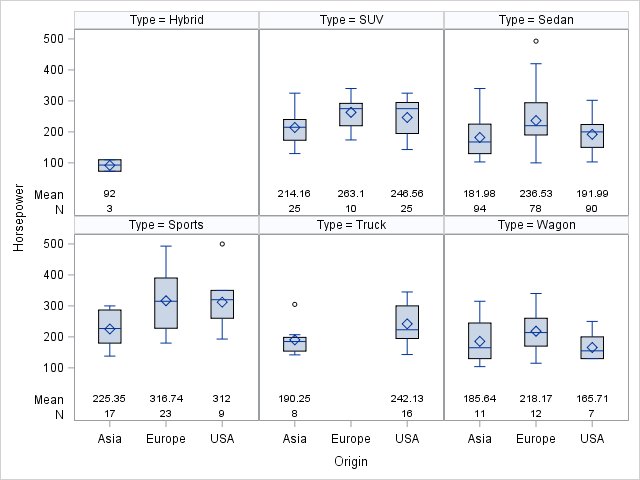## Adding Summary Statistics to VBOX SGPANEL

Hi SAS Community,

I only have SAS 9.4 TS1M4 and I'm trying to add the summary statistics to each panel, namely "N" and "Mean". I would like to do what the plot option DISPLAYSTATS does in TS1M5, but I'm having difficulty. I found a Graphically Speaking blog post showing how to do this in SGPLOT, but is there a way to do it with SGPANEL?

Here is my code:

``````Title1 'Mean HIV TNA PCR Ct Values by Category of Age Started ART';
Title2 'Categorized by Cohort';
proc sgpanel data=merged1;
where hivpcrct ne 0 ;
panelby cohort / rows=4 onepanel uniscale=column;
vbox hivpcrct / category = artstartcat ;
colaxis grid offsetmin=0.10 fitpolicy=rotatethin
VALUEATTRS=(Family=Times Size=10);
format cohort cohortf. artstartcat artstartcatf.;
RUN;title;``````

I want my plot to print the N and mean for each category of Age Started ART (artstartcat). I can use the "DATALABEL" plot option in VBAR, but not in VBOX.

Any help is appreciated!

Thanks,

Cara

1 ACCEPTED SOLUTION

Accepted SolutionsJay54
Meteorite | Level 14

## Re: Adding Summary Statistics to VBOX SGPANEL

As you say, the feature to add the statistics table to VBOX for SGPANEL was added in SAS 9.40M5.  The option uses the values already computed for the box plot.  To do the same with a prior SAS 9.4 release, you can use the ColAxisTable.  But, you will have to compute the statistics yourself using PROC MEANS and merge it into the data as shown below.

``````/*--Compute statistics by Type and Origin--*/
proc means data=sashelp.cars;
class type origin;
var horsepower;
output out=stat(where=(_type_ > 2))
mean=Mean N=N;
run;
proc print;run;

/*--Merge statistics with original data set--*/
data cars;
keep type origin horsepower mean n;
set sashelp.cars stat;
run;
proc print;run;

/*--Render VBox and statistics using ColAxisTable--*/
proc sgpanel data=cars;
panelby type;
vbox horsepower / category=origin;
colaxistable n mean;
run;``````2 REPLIES 2Jay54
Meteorite | Level 14

## Re: Adding Summary Statistics to VBOX SGPANEL

As you say, the feature to add the statistics table to VBOX for SGPANEL was added in SAS 9.40M5.  The option uses the values already computed for the box plot.  To do the same with a prior SAS 9.4 release, you can use the ColAxisTable.  But, you will have to compute the statistics yourself using PROC MEANS and merge it into the data as shown below.

``````/*--Compute statistics by Type and Origin--*/
proc means data=sashelp.cars;
class type origin;
var horsepower;
output out=stat(where=(_type_ > 2))
mean=Mean N=N;
run;
proc print;run;

/*--Merge statistics with original data set--*/
data cars;
keep type origin horsepower mean n;
set sashelp.cars stat;
run;
proc print;run;

/*--Render VBox and statistics using ColAxisTable--*/
proc sgpanel data=cars;
panelby type;
vbox horsepower / category=origin;
colaxistable n mean;
run;``````## Re: Adding Summary Statistics to VBOX SGPANEL

Thank you! This works nicely.

Discussion stats
• 2 replies
• 2954 views
• 1 like
• 2 in conversation